Multiplying with Zeros 1
(10 Questions)
California State Math Standards Included:
Grade 3
Number Sense
3.0 Students solve problems involving addition, subtraction, multiplication, and division of whole numbers and understand the relationships among the operations.
Multiply only by ten.
From Mr. Anker Tests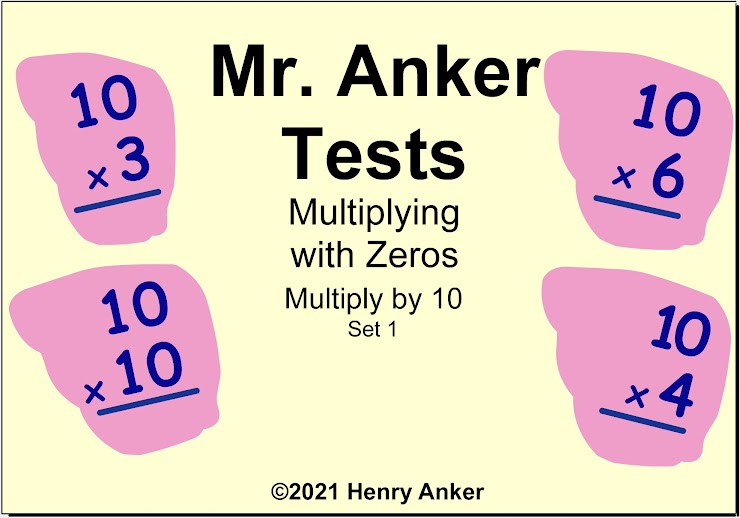1. Which of the choices below is the correct answer to this multiplication fact? *
1 point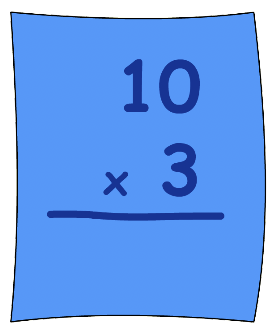2. In the space below, type the answer to this multiplication equation. *
1 point3. From the dropdown menu below, choose the correct answer to this multiplication fact. *
1 point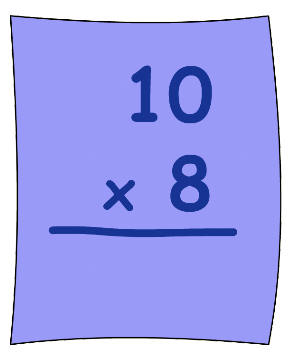4. In the space below, type the answer to this multiplication equation. *
1 point5. From the dropdown menu below, choose the correct answer to complete this multiplication fact. *
1 point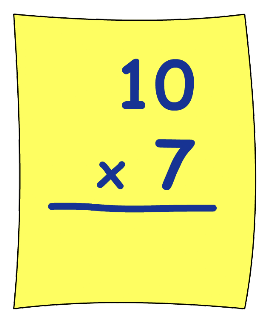6. Type your answer to this multiplication fact in the space below. *
1 point7. Type your answer to this multiplication fact in the space below. *
1 point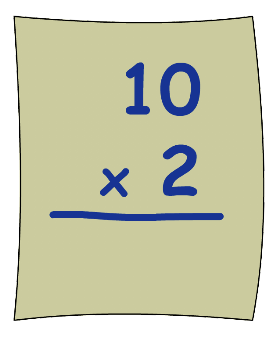8. Type your answer to this multiplication fact in the space below. *
1 point9. Choose the correct answer to this multiplication fact from the dropdown menu below. *
1 point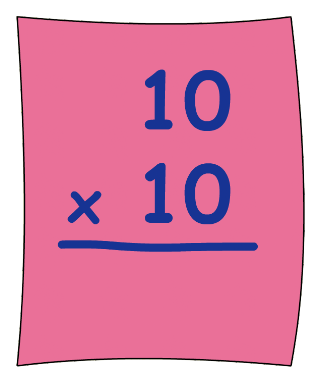10. Type your answer to this multiplication fact in the space below.
1 pointNever submit passwords through Google Forms.
This content is neither created nor endorsed by Google. Report Abuse - Terms of Service - Privacy Policy